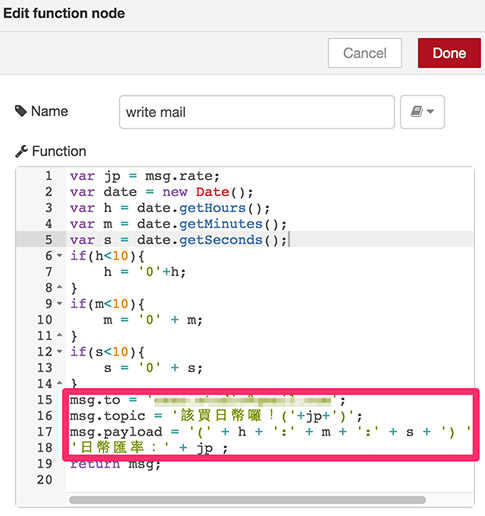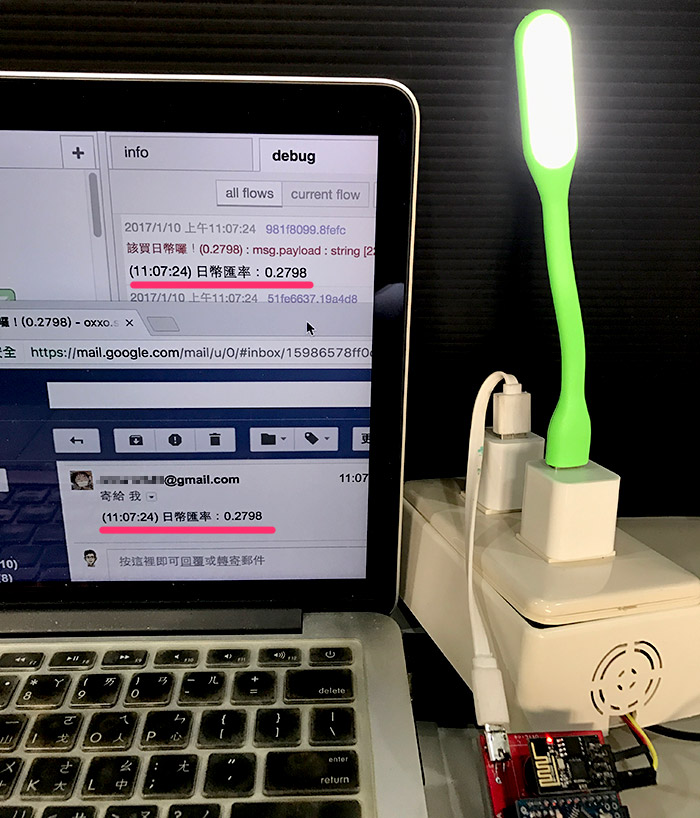2017 iT 邦幫忙鐵人賽
DAY 28
0
Modern Web

解析匯率網頁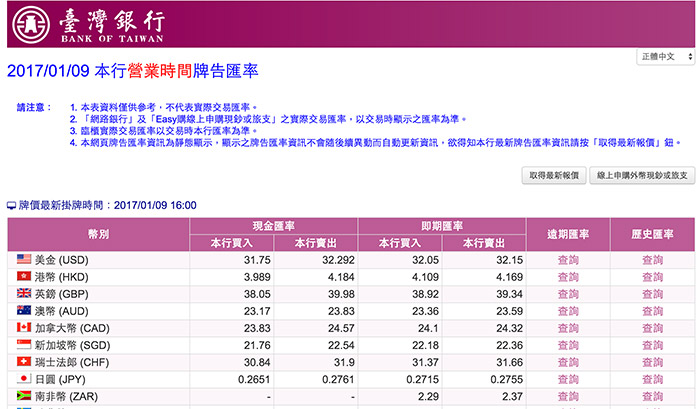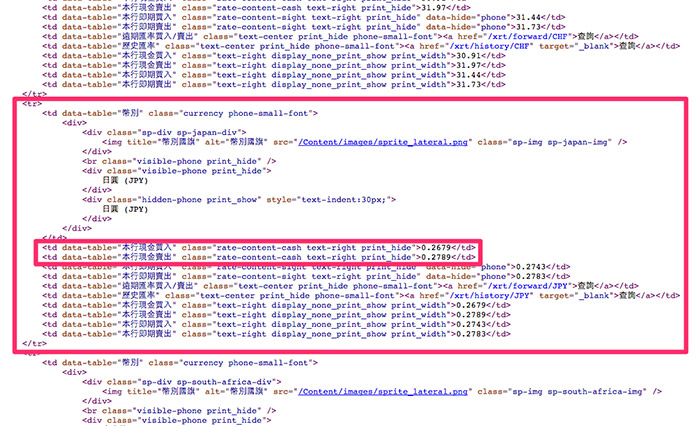rate-content-cash text-right print_hide使用 Node-RED 抓取匯率數值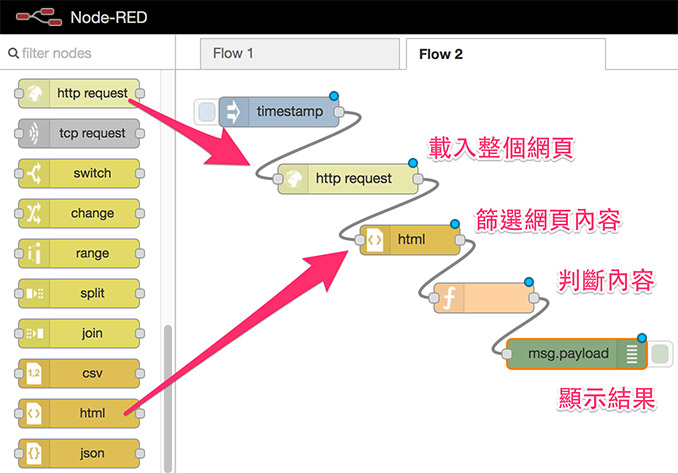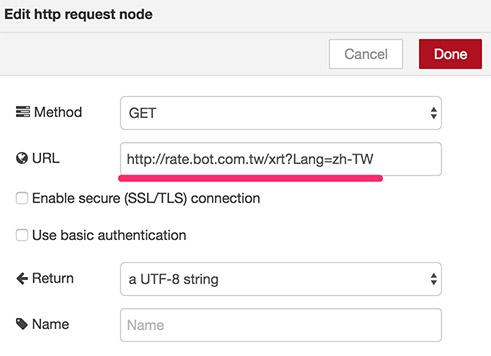Output 選擇 the html contentonly the text content 都可以，設定好之後可以賦予這個標籤一個名稱，我這邊給它命名為 filter。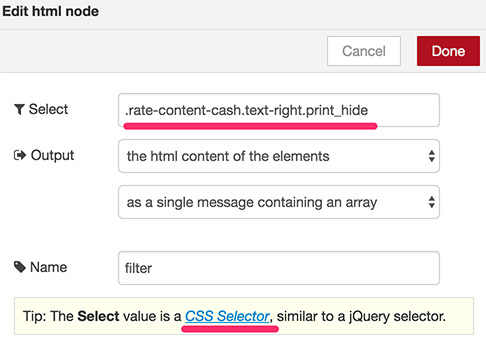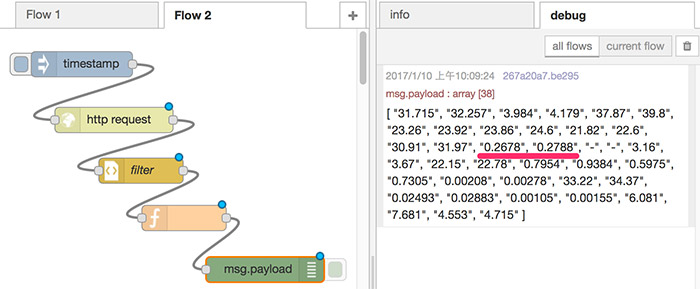var date = new Date();
var h = date.getHours();
var m = date.getMinutes();
var s = date.getSeconds();
if(h<10){
h = '0'+h;
}
if(m<10){
m = '0' + m;
}
if(s<10){
s = '0' + s;
}
msg.payload = '(' + h + ':' + m + ':' + s + ')\n'+
'日幣匯率：' + jp ;
return msg;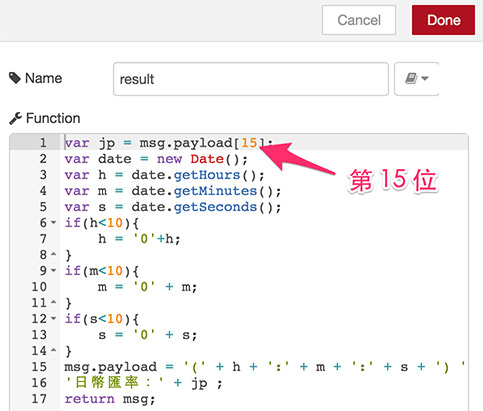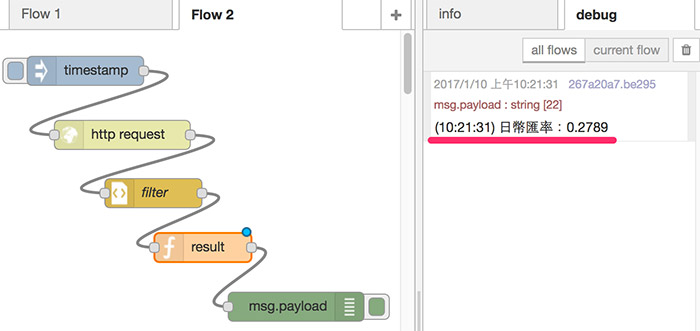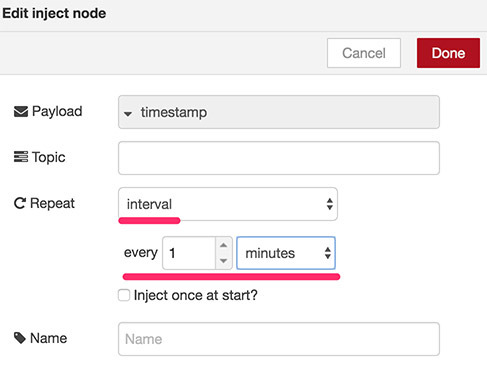到達匯率時，寄送 Email 並透過 Webduino 點亮燈泡msg.rate = jp;
if(jp<0.29){
}else{
}
return msg;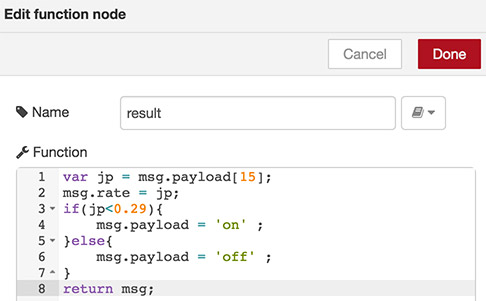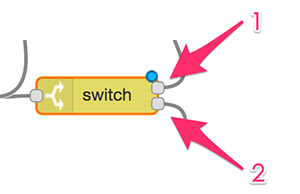var jp = msg.rate;
var date = new Date();
var h = date.getHours();
var m = date.getMinutes();
var s = date.getSeconds();
if(h<10){
h = '0'+h;
}
if(m<10){
m = '0' + m;
}
if(s<10){
s = '0' + s;
}
msg.to = 'xxxx@gmail.com';
msg.topic = '該買日幣囉！('+jp+')';
msg.payload = '(' + h + ':' + m + ':' + s + ') '+
'日幣匯率：' + jp ;
return msg;